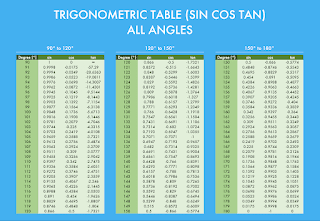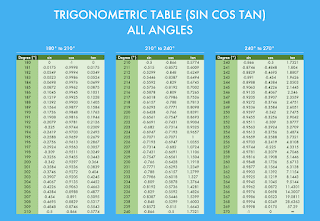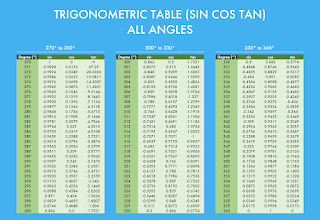# Trigonometric Table(Sin, Cos, Tan) From Angle 0 to 360 Degree

Trigonometric Table(Sin, Cos, Tan, Cosec, Sec & Cot) From Angle 0 to 360 Degree

In this post I have provided all the values of trigonometric ratios (sin, cos, tan, cosec, sec & cot) from angle 0 to 360 degree. These specific values of angle(0 to 360 degree) is very important for all the students because every student know only some value of specific angles like 0⁰, 30⁰, 45⁰, 60⁰ and 90⁰.

These specific angles are very important at the times of solving the numerical questions in the subject of physics and Chemistry. It values cannot be learn, because these are very complicated and different for all the trigonometrical ratios, that is why you can use these chart at the time of solving the numericals.

Given below are the trigonometrical ratios with their respective angles which can be noticed by the student.Trigonometric Table-2Trigonometric Table-3Trigonometric Table-4

Scroll to Top